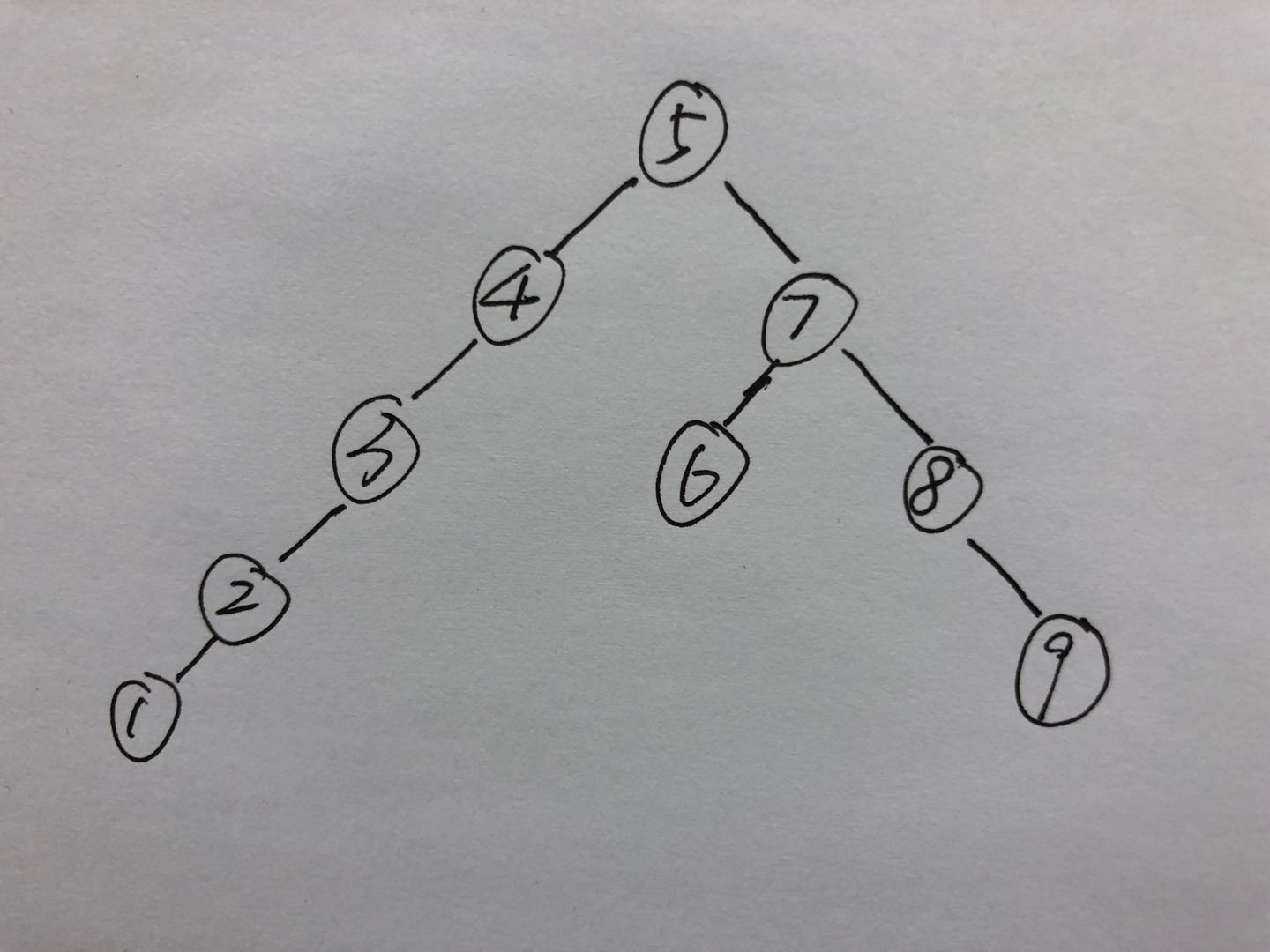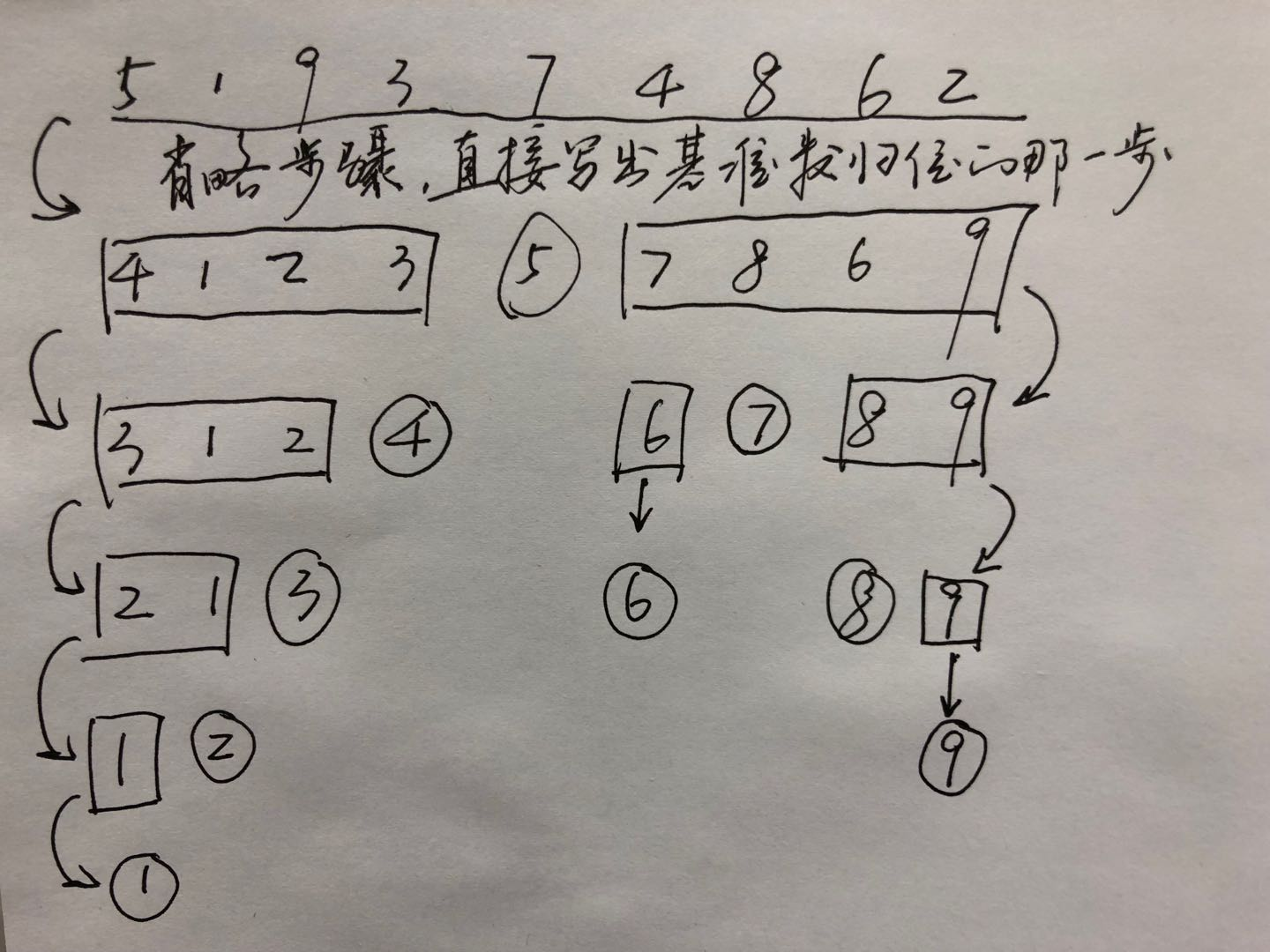﻿ 快速排序

### 快速排序

I)循环开始方向。

3 1 2 4 5
i->    <-j
3 1 2 4 5
i-> <-j
3 1 2 4 5
i->  j
3 1 2 4 5
i->j
3 1 2 4 5
ij

1）最终以i遇到j为终止标志

3 1 2 4 0 5
i->       <-j
3 1 2 4 0 5
i->    <-j
3 1 2 4 0 5
i-> <-j
3 1 2 4 0 5
i <-j

3 1 2 4 0 5
i  j
3 1 2 0 4 5
i j
3 1 2 0 4 5
i->j
3 1 2 0 4 5
ij
4 1 2 0 3 5
ij
2）最终以j遇到i为终止标志

3 1 2 4 5
i->    <-j
3 1 2 4 5
i-> <-j
3 1 2 4 5
i-><-j
3 1 2 4 5
i->j
3 1 2 4 5
i<-j
3 1 2 4 5
ij

4 1 2 3 5
ij

II)时间复杂度

``` 1 void quicksort(int *a,int left,int right){
2     int temp,index,i,j;
3     i=left;
4     j=right;
5     index=a[left];
6     if(left>right) return ;
7     while(i!=j){
8         while(a[j]>=index&&j>i){
9             j--;
10         }
11         while(a[i]<=index&&j>i){
12             i++;
13         }
14         if(i<j){
15             temp=a[i];
16             a[i]=a[j];
17             a[j]=temp;
18         }
19     }
20     a[left]=a[i];
21     a[i]=index;
22     quicksort(a,left,i-1);
23     quicksort(a,i+1,right);
24 }```1)最优时间复杂度。可以看到如果每次的分区都划分很均匀的话，那么我们可以得到最优情况的时间复杂度为T(n)，则有T(n)=2T(n/2)+n=4T(n/4)+2n=...=nT(1)+logn*n即为O(nlogn)。，
2)最坏时间复杂度。同理，最坏情况为正序或者反序，正序是形状的二叉树，反序则是/形状的二叉树，二者的时间复杂度T(n)=n-1+n-2+...+1=n(n-1)/2=O(n^2)。
3)平均复杂度。这个确实有点难算，暂时鸽了，等加强一下概率论的知识再来补全。